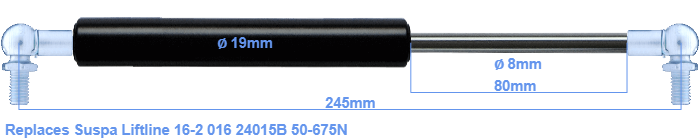# Replacement for Suspa Liftline 16-2 016 24015B 50-675N

\$35.06

Replacement gas spring for the Suspa Liftline 16-2 016 24015B 50-675 Newton. This fits a (possibly already present) ball with a diameter of 10mm. A corresponding ball is included. Brand: Stabilus Industry Line.
 Force Choose an option50 Newton60 Newton80 Newton100 Newton120 Newton140 Newton150 Newton160 Newton180 Newton200 Newton220 Newton240 Newton250 Newton260 Newton280 Newton300 Newton320 Newton340 Newton350 Newton360 Newton380 Newton400 Newton420 Newton440 Newton450 Newton460 Newton480 Newton500 Newton520 Newton540 Newton550 Newton560 Newton580 Newton600 Newton620 Newton640 Newton650 Newton660 Newton675 NewtonClearThe diameter of the cylinder of this gas spring is 19 millimeters. The diameter of the rod totals 8 mm. The stroke is 80 millimeter long. The total length of this gas strut is 245 mm. This is the distance between the centers of the mounting parts. Without the mounting parts this gas spring is 205 mm long (thread to thread). The force of this replacement gas spring is 50-675 Newton. This is not an official Suspa Liftline gas spring, but a so called replacement. This replacement gas spring is a Stabilus Industry Line gas spring. The gas spring can be used without problems as a replacement, because the dimensions and force equal.
Category: